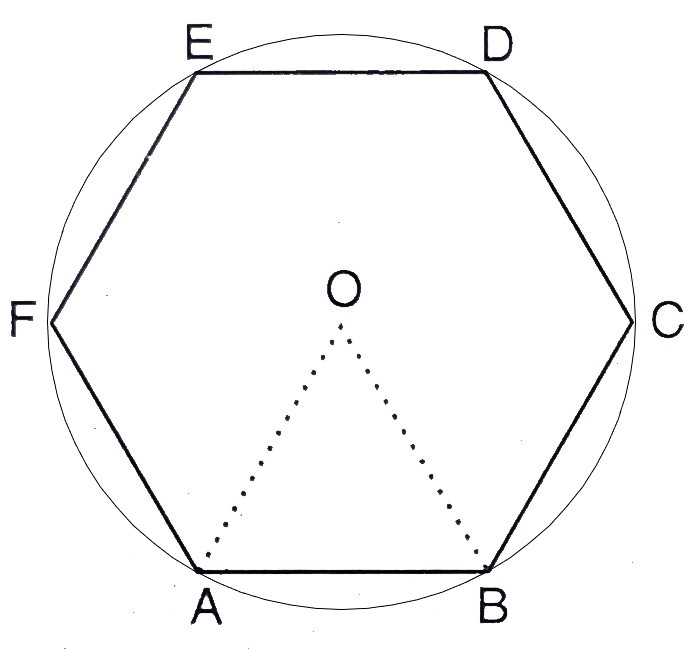# A regular hexagon is inscribed in a circle. If the area of hexagon is $24 \sqrt{3} \mathrm{~cm}^{2}$, find the area of the circle. (Use $\pi=3.14$ )

Given:

A regular hexagon is inscribed in a circle.

The area of the hexagon is $24 \sqrt{3} \mathrm{~cm}^{2}$.

To do:

We have to find the area of the circle.

Solution:Let $ABCDEF$ be the regular hexagon and $O$ is the centre.

We get six equal equilateral triangles by joining the vertices of the hexagon with $O$.

Let the radius of the circle $=$ Length of the side of the equilateral triangle $AOB=r$

Area of the hexagon $=24\sqrt{3}\ cm^2$

This implies,

$6\times\frac{\sqrt{3}}{4} r^{2}=24\sqrt{3}$

$\Rightarrow r^{2}=\frac{24\sqrt{3}\times 4}{6\sqrt{3}}$

$\Rightarrow r^{2}=16$

Area of the circle $=\pi r^{2}$

$=3.14 \times 16$

$=50.24 \mathrm{~cm}^{2}$

The area of the circle is $50.24 \mathrm{~cm}^{2}$.

Updated on: 10-Oct-2022

119 Views